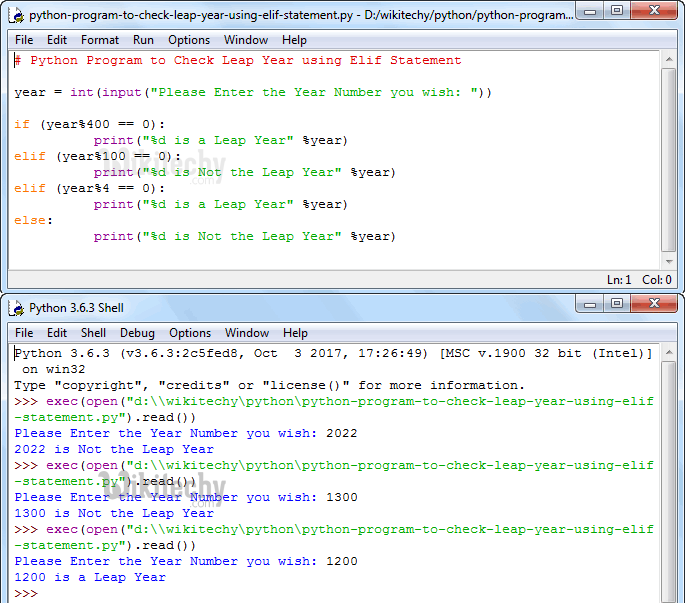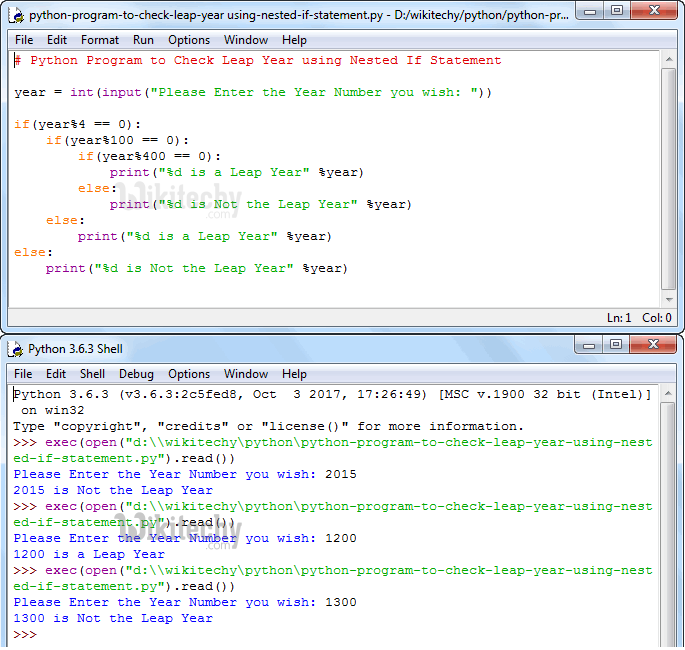# python tutorial - Python Program to Check Leap Year - learn python - python programming

• To write Python Program to Check Leap Year or Not using If Statement, Nested If Statement and Elif Statement in Python with example.
• Before we get into programs, Let us see the logic and definition behind the Leap YearLearn Python - Python tutorial - Python Program to Check Leap Year - Python examples - Python programs

## Leap year

• The normal year contains 365 days but the leap year contains 366 days. Logically, All the years that are perfectly divisible by 4 are called as Leap years except the century years. Century year’s means they end with 00 such as 1200, 1300, 2400, 2500 etc (Obviously they are divisible by 100). For these century years we have to calculate further to check the Leap year.
• If the century year is divisible by 400 then that year is a Leap year
• If the century year is not divisible by 400 then that year is a Leap year

## Python Program to Check Leap Year using If Statement

• This program allows the user to enter any year and then it will check whether the user entered year is Leap year or not using the Python If statement.

## OutputLearn Python - Python tutorial - Python Program to Check Leap Year using If Statement - Python examples - Python programs

• Let us check for Leap Year and normal year (Not Leap Year)

## Analysis

• Since we have to check multiple conditions within one If Statement, we used Logical AND and Logical OR operators. Let us divide the condition to understand it better
• First condition (year%400 == 0) will check whether the (year%400) reminder is exactly equal to 0 or not. As per the algorithm any number that is divisible by 400 is a Leap year.

## OR

• Second condition holds 2 statements with Logical AND operator, so they both have to be TRUE.
• First condition (year%4 == 0) will check whether the reminder of the (year % 4) is exactly equal to 0 or not. If the condition is False then it will exit from the condition because there is no point in checking the other condition. It is definitely not the Leap year. AND
• Second condition will check (year % 100) reminder is not equal to 0. If it is TRUE then the given number is not century number.
• As per the algorithm any number that is divisible by 4 but not divisible by 100 then that number is Leap Year.

TIP: Please refer Python Logical Operators to understand the functionality of Logical And and Logical Or.

## Python Program to Check Leap Year using Elif Statement

• This program allows the user to enter any year and then it will check whether the user entered year is Leap year or not using the Python Elif Statement.

## OutputLearn Python - Python tutorial - Python Program to Check Leap Year using Elif Statement - Python examples - Python programs

## Analysis

• User will enter any year to check whether that year is Leap year or Not.
• First If condition will check whether the (year % 400) reminder is exactly equal to 0. As per the algorithm any number that is divisible by 400 is a Leap year. If this condition Fails then it will go to next condition.
• Second If condition will check (year % 100) reminder is exactly equal to 0 or not. As per the algorithm any number that is not divisible by 400 but divisible by 100 is Not a Leap year (Century Year). We checked (year % 400) in the First If statement. Because it is failed it came to second condition. If both the first and second condition Fails then it will go to third condition.
• Third condition will check whether year mod 4 is equal to 0. If this condition is True then given year is Leap year because We already checked for the century years in the previous condition. If all the statements Fails then it will go Else statement at the end.
• If all the above statements fail then it is not Leap year

## Python Program to Check Leap Year using Nested If Statement

• This program allows the user to enter any year and then it will check whether the user entered year is Leap year or not using the Python Nested If statement.

## OutputLearn Python - Python tutorial - Python Program to Check Leap Year using Nested If Statement - Python examples - Python programs

## Analysis

• User will enter any year to check whether that year is Leap year or Not. First If condition will check whether the reminder of the (year%4) is exactly equal to 0 or not.
• If the condition is False then the given number is definitely not the Leap year.
• If the condition is True then we have check further for the century year. So the compiler will go to the Nested If condition.
• Second If condition will check (year%100) reminder is exactly equal to 0 or Not.
• If this condition is False then the year is not century year. So the given number is definitely Leap year.
• If the condition is True then we have check whether the number is divisible by 400 or not. So the compiler will goto to another Nested If condition.
• In this condition, compiler will check whether the remainder of the (year%400) is exactly equal to 0 or not.
• If the condition is False then the given number is definitely not the Leap year.
• If the condition is True then the given number is Leap Year